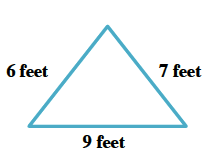### Home > MC1 > Chapter 2 > Lesson 2.2.2 > Problem2-70

2-70.

A triangular flower bed (area for planting flowers) needs a thin metal border all the way around it. The sides are $7$ feet, $6$ feet, and $9$ feet long. Homework Help ✎

1. How many feet of border should be purchased? Make a sketch and show your work.

This problem is asking you to find the perimeter (distance around) the flower bed. Refer to problem 2-47 if you need help before looking at the solution.

Begin by sketching a picture of the flower bed with labels.

Add the lengths of the sides to find the perimeter.

$7$ feet + $6$ feet + $9$ feet = $22$ feetIn order to cover the perimeter, $22$ feet of border should be purchased.

1. If borders cost $\8.75$ per yard (and only whole numbers of yards can be purchased), how much would the border cost?

Remember to convert the length of border to yards before finding the cost.